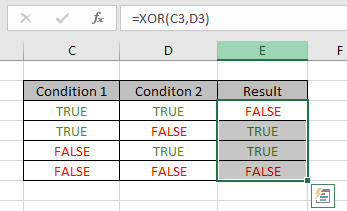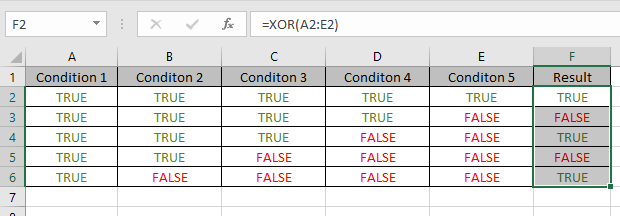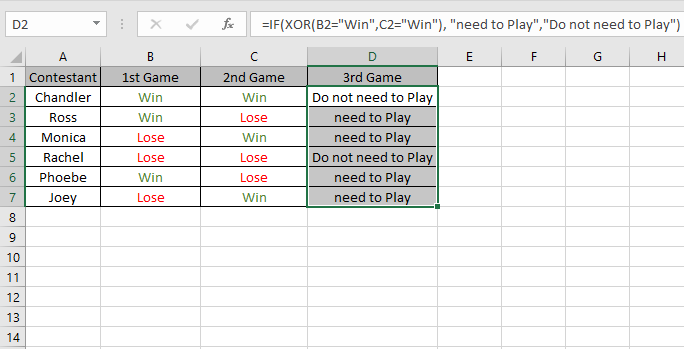# How to use the XOR function in Excel

In simple words, logic_test are performed in excel to perform an action based on the condition stands True or False. XOR function is an exclusive OR function which checks multiple conditions and returns the result of logic_test.

The XOR function returns the result of exclusive OR applied on conditions.
Syntax:

=XOR (logical1, [logical2], ...)

Logical1 : first logic test
Here are the results of XOR function performed on 2 conditions.

Use the formula:

=XOR(C3,D3)As you can see the XOR function returns TRUE if there are odd count of TRUEs else it returns False.

Here are the results of XOR function performed on 5 conditions.Use the formula:

=XOR(A2:E2)

A2:E2 : multiple values can be given as an array argument
The formula drops down to
=XOR({TRUE,TRUE,TRUE,TRUE,TRUE})
This all might be confusing to you. Let’s gear up & start understanding this function using it in an example.

Here we have Some friends who are playing games and they have one rule. Best of Three to qualify for the next round, this means a Player doesn’t require to play the 3rd match if the player lost the first two games or won the first two games. If the person lost the first two games, he or she is out of competition and If the person won the first two games qualifies directly to the next round. Remaining others need to play the third game to decide who will qualify for further rounds.Use the formula with the IF function

=IF (XOR (B2 = "Win", C2 = "Win"), "need to Play", "Do not need to Play")As you can see from the above example XOR function in excel
Notes:

• Logical arguments must evaluate to TRUE or FALSE, 1 or 0, or references that contain logical values.
Empty references are ignored.
• XOR returns #VALUE! error if no logical values are found.
• With more than two logicals, XOR returns TRUE when the number of TRUE logicals is odd, or else it returns FALSE.

Hope you understood how to use XOR function and referring cell in Excel. Explore more articles on Excel function here. Please feel free to state your query or feedback for the above article.

Related Articles:

How to use the Excel OR function

How to use the Excel AND Function

IF with AND Function in Excel

How to use the Excel TRUE Function

How to use the Excel NOT function

IF not this or that in Microsoft Excel

IF with AND and OR function in Excel

Popular Articles:

50 Excel Shortcuts to Increase Your Productivity

How to use the VLOOKUP Function in Excel

How to use the COUNTIF in Excel 2016

How to use the SUMIF Function in Excel

Terms and Conditions of use

The applications/code on this site are distributed as is and without warranties or liability. In no event shall the owner of the copyrights, or the authors of the applications/code be liable for any loss of profit, any problems or any damage resulting from the use or evaluation of the applications/code.